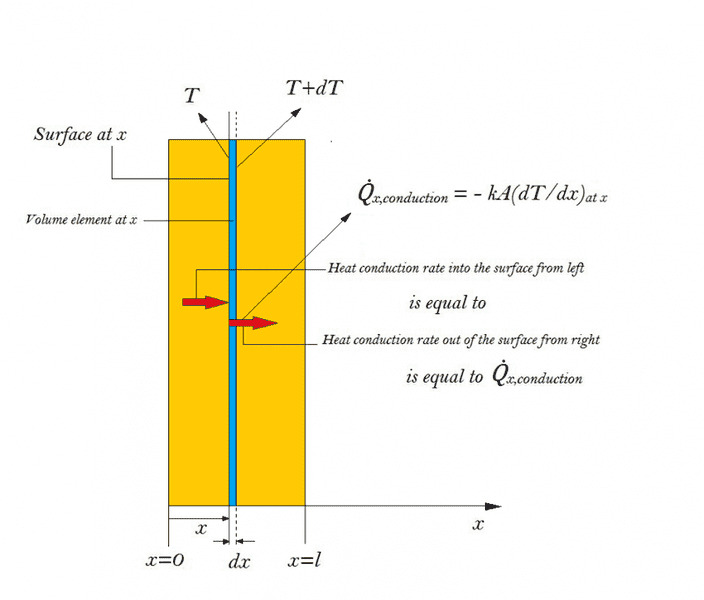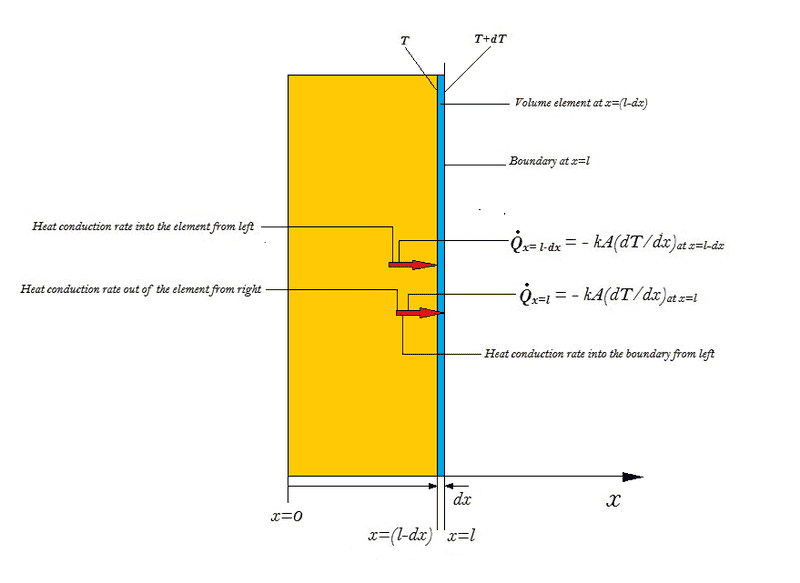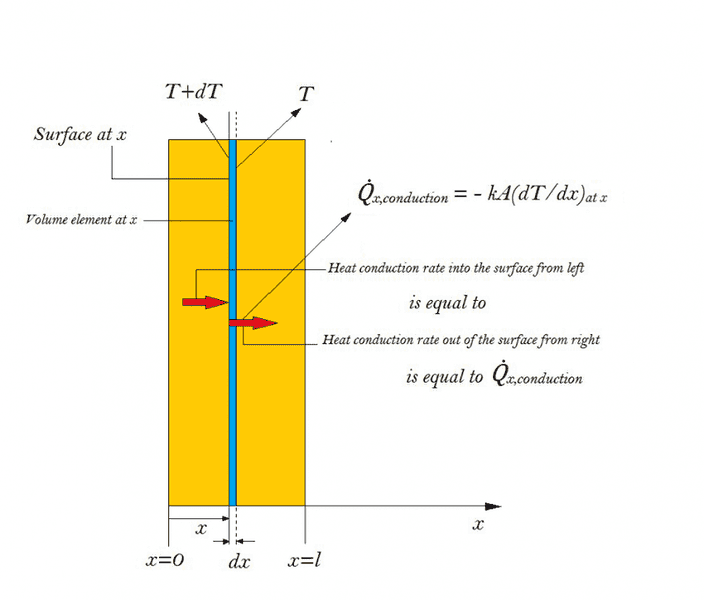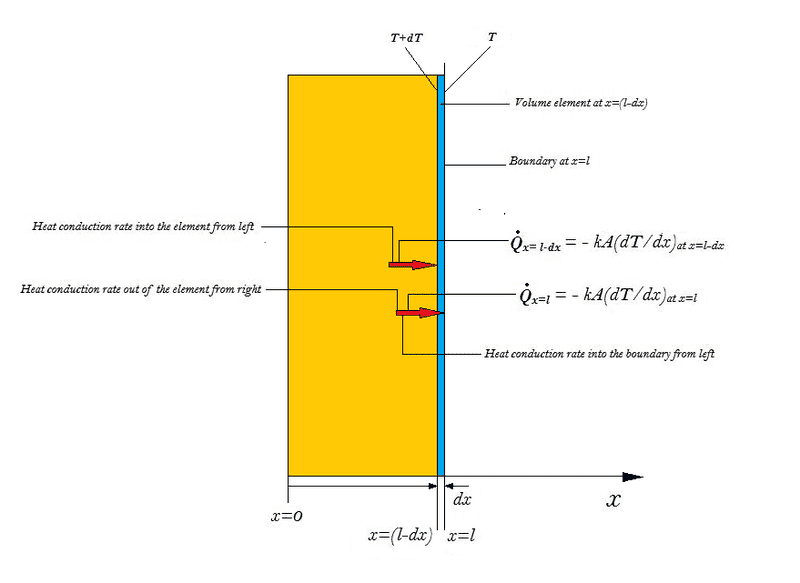# Fourier's Law of Heat Conduction

Considering one dimensional heat conduction we may write the Fourier's law of heat conduction in the x-direction as,

##\dot{Q}_{x}=-kA\Big(\frac{∂T} {∂x}\Big)##

where, ##\frac{∂T} {∂x}## is the temperature gradient which is basically the slope of the temperature curve on a T-x diagram.

Referring to the first attachment, if we consider a differential volume element at a distance 'x' from the origin of length 'dx' and cross sectional area 'A' normal to the direction of heat transfer, the rate of heat conduction through the left face of the element (the surface at 'x') is given by Fourier's law as,

##\dot{Q}_{x}=-kA \Big(\frac{∂T} {∂x}\Big)##

where,##\frac{∂T} {∂x}## is the temperature gradient 'ahead' of the surface at 'x' or it is the rate of change of temperature with the spatial coordinate 'x' for an infinitesimal change in the spatial coordinate as 'dx',the change being measured from the right of the surface at 'x' (the right hand derivative of 'T' with respect to 'x' at location 'x').

So we observe the rate of heat conduction through a surface is proportional to the temperature gradient immediately 'ahead' of the surface.

I have been observing some of the boundary conditions for heat transfer problems where they are applying Fourier's law to calculate the heat conduction rate (or sometimes heat flux) at the boundaries of a body (a slab of a material).

Referring to the second attachment, we again consider a differential volume element immediately adjacent to the boundary surface at x=l of thickness 'dx' and area 'A' normal to the direction of heat transfer.As we might observe if we wish to calculate the rate of heat conduction through the boundary surface at x=l, it might be written according to Fourier's law as,

##\dot{Q}_{x=l}=-kA \Big(\frac{∂T} {∂x}\Big)_{x=l}##

where, ##\Big(\frac{∂T} {∂x}\Big)_{x=l}## should be the temperature gradient 'ahead' of the surface at 'x=l'.But in this case the temperature curve ends at the boundary i.e, at x=l within the medium itself.

So how are we supposed to visualize the slope of the temperature curve on the T-x diagram i.e, the temperature gradient at location 'x=l' ?BvU
Homework Helper
Actually the partial derivative is not localized 'ahead' or 'behind'. ##dx## is usually simply a limit for ##\Delta x \downarrow 0## for continuous functions.

Your question is more about functions being continuously differentiable, right-differentiable (##\Delta x > 0## & ##\Delta x \downarrow 0## ), or left-differentiable (##\Delta x < 0## & ##\Delta x \uparrow 0## ) .

If k changes abruptly, you have a discontinuity in ##\partial T\over \partial x##, that's all.

•Soumalya
Actually the partial derivative is not localized 'ahead' or 'behind'. ##dx## is usually simply a limit for ##\Delta x \downarrow 0## for continuous functions.

Your question is more about functions being continuously differentiable, right-differentiable (##\Delta x > 0## & ##\Delta x \downarrow 0## ), or left-differentiable (##\Delta x < 0## & ##\Delta x \uparrow 0## ) .

Yes I agree 'dx' implies the limit of Δx → 0,so at the boundary surface i.e, at x=l if we take a Δx it will be outside the medium and even if we take the limit of Δx → 0 , it will still be outside the medium.

Well while taking derivatives of a function we can either take a right hand derivative or a left hand derivative at any location 'x'.I am not taking about the continuity of the temperature curve rather I meant that since according to fourier's law the rate of heat conduction is proportional to the very small change in temperature 'dT' in the direction of heat conduction over a very small change in distance 'dx' and is inversely proportional to 'dx', we calculate the rate of change of temperature with distance at the location 'x' by taking a small increment in distance Δx and recording the small change in temperature 'ΔT' ,later taking the limit of this increment to an infinitesimal amount (Δx → 0).

So if we take a Δx at x=l it will be ahead of x=l which lies outside the boundary of the medium.The temperature curve however is up to the boundary of the medium.Then how do we calculate ##\Big(\frac{∂T} {∂x}\Big)_{x=l}## ?

If k changes abruptly, you have a discontinuity in ##\partial T\over \partial x##, that's all.

I am not sure what was meant by the statement.Can you elucidate further?

BvU
Homework Helper
So if we take a Δx at x=l it will be ahead of x=l which lies outside the boundary of the medium.The temperature curve however is up to the boundary of the medium.Then how do we calculate ##
\Big(\frac{∂T} {∂x}\Big)_{x=l}## ?
At such a point we can only take the left derivative (completely inside the 'yellow' material in your picture). It will not have the same value as the right derivative (that is completely inside the 'next' material). But you do have $$\dot{Q}_{x}=-k_{\rm \, left} A \Big(\frac{∂T} {∂x}\Big)_{\rm left} =-k_{\rm \, right} A \Big(\frac{∂T} {∂x}\Big)_{\rm right}$$

In other words: If k changes abruptly, you have a discontinuity in ##\, \frac{∂T} {∂x}##.

By the way: you have the same issue at ##x=0## !

--

•Soumalya
At such a point we can only take the left derivative (completely inside the 'yellow' material in your picture). It will not have the same value as the right derivative (that is completely inside the 'next' material). But you do have $$\dot{Q}_{x}=-k_{\rm \, left} A \Big(\frac{∂T} {∂x}\Big)_{\rm left} =-k_{\rm \, right} A \Big(\frac{∂T} {∂x}\Big)_{\rm right}$$

In other words: If k changes abruptly, you have a discontinuity in ##\, \frac{∂T} {∂x}##.

By the way: you have the same issue at ##x=0## !

--

Yes your argument seems to be apparently correct.But I am confused by the fact that while calculating the rate of heat conduction through the boundary surface at x=l shouldn't we consider only the right hand derivative of temperature with respect to the spatial coordinate i.e,
$$\Big(\frac{∂T} {∂x}\Big)_{\rm right_{at x=l}}$$
?

If we consider the surface at x=l-dx (referring to the second attachment) the rate of heat conduction through the surface is also given by,

$$\dot{Q}_{x=l-dx} =-k_{material} A \Big(\frac{∂T} {∂x}\Big)_{\rm right_{at x=l-dx}}$$

If we assume the rate of heat conduction at the boundary (i.e, at x=l) to be,

$$\dot{Q}_{x=l} =-k_{material} A \Big(\frac{∂T} {∂x}\Big)_{\rm left_{at x=l}}$$

we will have $$\dot{Q}_{x=l-dx}$$ = $$\dot{Q}_{x=l}$$ since $$\Big(\frac{∂T} {∂x}\Big)_{\rm right_{at x=l-dx}}$$=$$\Big(\frac{∂T} {∂x}\Big)_{\rm left_{at x=l}}$$ i.e, the right hand derivative of temperature with respect to 'x' at 'x=l-dx' is equal to the left hand derivative of temperature with respect to 'x' at 'x=l'.

But $$\dot{Q}_{x=l-dx}$$ = $$\dot{Q}_{x=l}$$ during a steady heat conduction process only and if we are considering a transient heat transfer problem we must have
$$\dot{Q}_{x=l-dx}$$ ≠ $$\dot{Q}_{x=l}$$

So taking the left hand derivative of temperature at 'x=l' seems to be contradictory while evaluating $$\dot{Q}_{x=l}$$.Am I making a mistake somewhere?Chestermiller
Mentor
Where did you get these figures from? Did you develop them yourself, or are they out of some textbook?

Before you start looking at the situation where there is a discontinuity in the material at a boundary, you first need to understand how to handle a continuous medium.

Chet

•Soumalya
Where did you get these figures from? Did you develop them yourself, or are they out of some textbook?

Chet

These figures were developed by me to illustrate my query pictorially.I noticed I made a mistake the temperatures of the faces of the volume element were incorrectly marked.They should be interchanged.

Before you start looking at the situation where there is a discontinuity in the material at a boundary, you first need to understand how to handle a continuous medium.

Chet

It would of great help if you could present to me the matter with the prerequisites necessary before attempting to resolve my confusion.

Am I able to give a rough impression of where do I face a doubt?

These are the figures with those corrections.Chestermiller
Mentor
I don't like the figures at all. The heat balance should start off with the steady state heat balance equation:

$$Q]_{x+Δx}-Q]_x=0$$
where Q is the rate of heat flow.
If I divide this by Δx, and take the limit as Δx approaches zero, I obtain:
$$\frac{dQ}{dx}=0$$This is called the differential heat balance equation.

Now, the local rate of heat flow at location x is given by:
$$Q(x)=-kA\frac{dT}{dx}$$
If I substitute this into the differential heat balance equation, I get:
$$\frac{d}{dx}\left(kA\frac{dT}{dx}\right)=0$$

•Soumalya and BvU
I don't like the figures at all. The heat balance should start off with the steady state heat balance equation:

$$Q]_{x+Δx}-Q]_x=0$$
where Q is the rate of heat flow.

We might generalize our heat conduction equation to be fit for the case of transient heat conduction.

During transient conduction we have, $$Q]_{x+Δx}-Q]_x≠0$$

Now, the local rate of heat flow at location x is given by:
$$Q(x)=-kA\frac{dT}{dx}$$

If I understand it correctly the term $$\frac{dT}{dx}$$ is the local temperature gradient at location 'x'.Mathematically this is the derivative of temperature with respect to 'x' at location 'x'.Now,we might consider either the right hand derivative or the left hand derivative of 'T' with respect to 'x' at location 'x'.At this point I would like to clarify if the term $$\frac{dT}{dx}$$ in the Fourier's law can be taken as either the right hand or the left hand derivative of 'T' with respect to 'x' at location 'x'.

Within the medium if the temperature is a continuous and differentiable function in 'x' and we must have both the left hand and right hand derivatives equal in magnitude.But what if we wish to use Fourier's law at a boundary location where we are dealing with a discontinuity?

I don't like the figures at all

Why are the figures invalid?

Chestermiller
Mentor
Within the medium if the temperature is a continuous and differentiable function in 'x' and we must have both the left hand and right hand derivatives equal in magnitude.But what if we wish to use Fourier's law at a boundary location where we are dealing with a discontinuity?
At a boundary between two media with different properties, we have continuity of the temperature and of the heat flux. So, if the boundary is at x = L, then
$$T]_{x=L^+}=T]_{x=L^-}$$
$$\left[k\frac{dT}{dx}\right]_{x=L^+}=\left[k\frac{dT}{dx}\right]_{x=L^-}$$
where the superscripts + and - represent the values on the two sides of the boundary

I really don't want to go into what I don't like about the diagrams, since its just a matter of personal taste.

Chet

•Soumalya